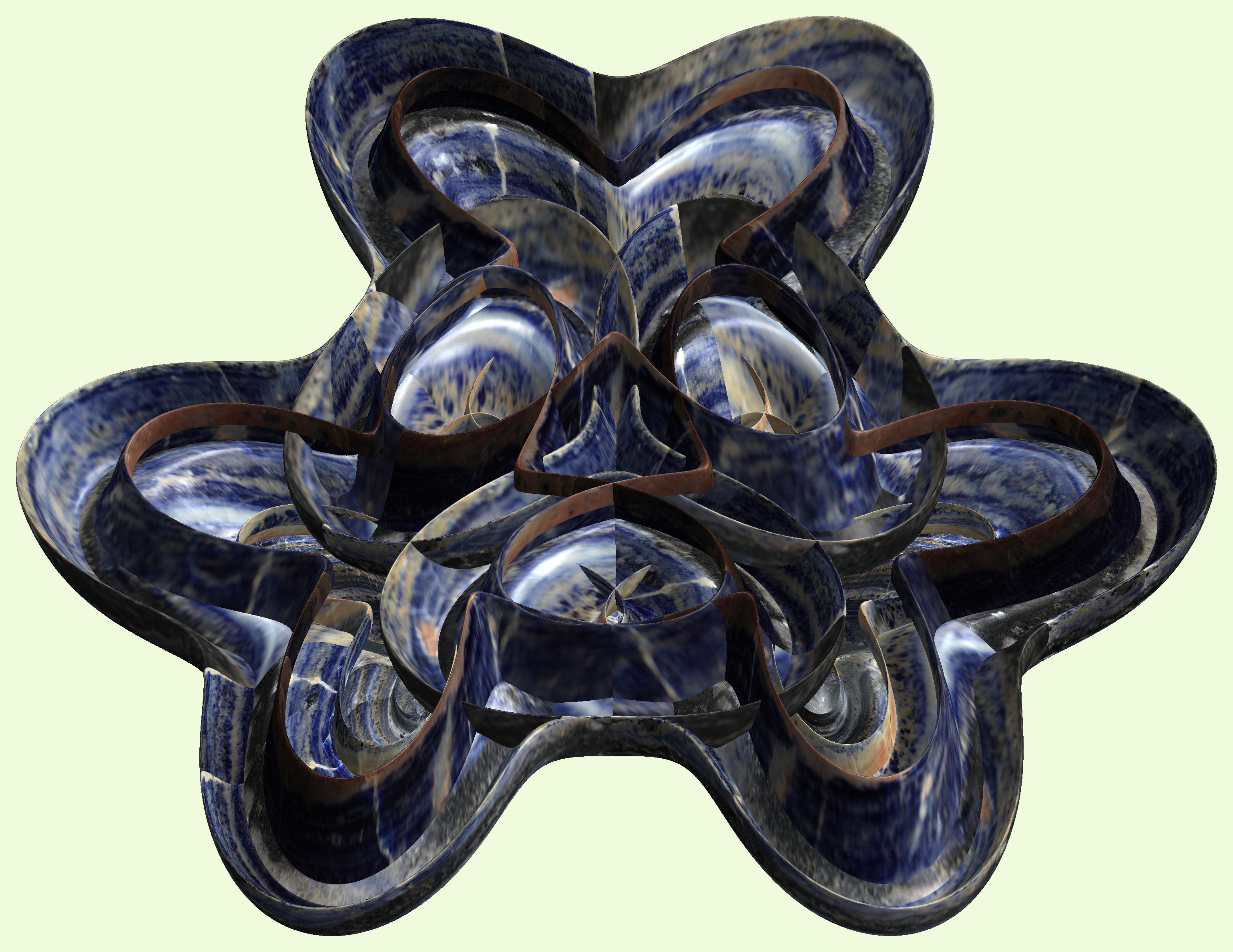3 Cups / ３個のカップ3 cups / ３個のカップ free stock photo

(* === Following code of Mathematica 8 generates this image. === *)

a = 6; (* center hole size of a torus *)

b1 = 4; (* the number of angles *)

b2 = 3; (* the number of waves *)

b3 = 1; (* the number of knots *)

c = 80; (* distance from the center of rotation *)

d = 3; (* the number of tori *)

h1 = 2; (* width of a torus *)

h2 = 2; (* width of a torus *)

h3 = 1; (* height of a torus *)

SetOptions[ParametricPlot3D,

PlotRange -> Full, Mesh -> None, Boxed -> False, Axes -> None,

PlotPoints -> 600, ImageSize -> 3000, Background -> RGBColor[{240, 250, 220}/255],

PlotStyle -> Directive[Specularity[White, 90], Texture[Import["D:/tmp/94.jpg"]]],

TextureCoordinateFunction -> ({#4, #5 Pi} &), Lighting -> "Neutral"];

g[v_] := Cos[2 Cos[Cos[Cos[v]]]];

x = t (a - h1 Cos[t] + h2 Sin[b1 s]) Cos[b3 (s + Pi/(2 b1))] + c;

y = t (a - h1 Cos[t] + h2 Sin[b1 s]) Sin[b3 (s + Pi/(2 b1))];

z = t (a - h3 (g[t] + Sin[b2 t]));

rm = Table[{x, y, z}.RotationMatrix[2 i Pi/d, {0, 0, 1}], {i, d}];

ParametricPlot3D[rm, {t, 0, 6 Pi}, {s, 0, 2 Pi}]

(*---

Another shapes and colors, and the meaning of this code in the set description

*)

Free high resolution images 3 cups / ３個のカップ, グラフィクス, グラフィック, コード, シンメトリー, アルゴリズム.
Licence: Creative Commons - CC0. Photos transferred to the public domain from Creative Commons.

Tags: